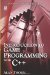2.4 Algebraic Expressions

2.4 Algebraic Expressions

Having considered some of the rules and ideas used in algebra such as negative numbers, exponents, and properties of multiplication and division, it is time to examine some simple algebraic expressions where letters are used in place of numbers. The letters represent numbers that we do not know. Consider the following expression:

• 2a + 5a + 7a 9a

 Note Please note that in most algebra multiplication, the cross symbol is omitted and the two factors are written side by side.

The above expression has four terms: 2a, 5a, 7a, and 9a. Terms are separated by addition and subtraction symbols. Each term has two factors; for example, 2a means 2 × a. Both 2 and a are factors. The letter a represents an unknown number, and is called a variable or literal.

2.4.1 Coefficients

In the factors 2a, 2 is said to be the coefficient of a, and a is said to be the coefficient of 2. In the factors abcd (which means a × b × c × d), the coefficient of a is bcd and the coefficient of b is acd. Therefore, a coefficient of any factor is every other factor (not including itself) in a single term.

2.4.2 Like Terms

In an algebraic expression, any two terms are said to be like terms when they have the same variable factors; in other words, they may have different actual number values but they will be multiplied by the same letters.

• 2a and 3a are like terms because both terms are multiplied by a.

• 4ab and 7ab are like terms because ab is a common factor.

• 7a and 3b are not like terms because they do not share the same variable factor.

• 9a and 9ab are not like terms because a and ab are different factors.

• 7abcd and 9bcda are like terms because multiplication is commutative, meaning that a × b × c × d is the same as b × c × d × a. (The order of multiplication does not matter.) Factors are usually written in alphabetical order.

• 7ab × 9cd appear as though they are separate terms, but this is in fact a single term and each of these numbers and variables is a factor of the single term. Only addition and subtraction separates terms. This term therefore should be rewritten more clearly as 63abcd.

2.4.3 Collect Like Terms

In algebra, subtraction is seen as adding a negative number. We know that 5 + (3) is the same as 5 3 (the minus sign belongs to 3; minus is the sign and 3 is the absolute value). The previous section demonstrated how like terms can be considered those with common variable factors, such as 2a and 5a. Whenever like terms are encountered, they can be added together to form a single term.

• 2a + 5a + 7a = 14a

• 7ab 2ab = 5ab

• 5x + 2x + 5y 2y = 7x +3yIntroduction to Game Programming with C++ (Wordware Game Developers Library)
ISBN: 1598220322
EAN: 2147483647
Year: 2007
Pages: 225
Authors: Alan Thorn

Similar book on Amazon